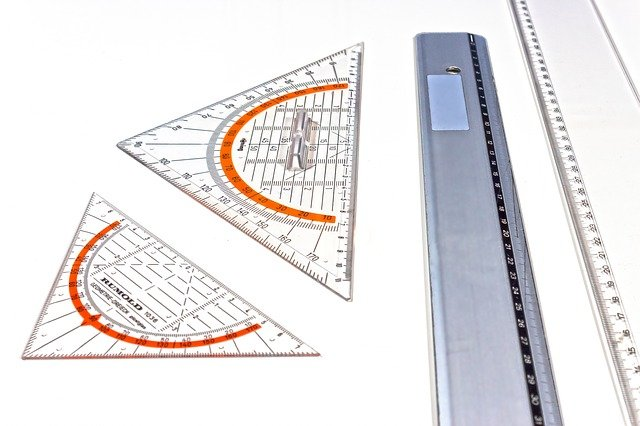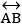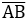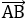# Lines and Angles Class 9th

## Introduction

In the previous classes, we studied line segments, rays, and lines and their properties. We also know the difference between them. Now, we shall study lines and angles, the formation of angles with the help of lines, theorems based on the properties, etc.

We also know the importance of lines and angles in our daily life. In our houses, examples of lines are iron or steel rods in the windows or doors, different types of linear designs and examples of angles are angles made between walls and floor, the angle made between walls and ceiling. Let us recall the definitions studied in the previous classes which are related to lines and angles.

### Some Definitions

Line – The combination of many points which are arranged in a flow with almost negligible width and depth is called the Line. The line has no endpoint so it can be increased in both directions. Line AB is denoted by. Sometimes we use small letters like l, m, and n to denote lines.

Line Segment – The fixed portion of a line that has two endpoints and cannot be increased in any direction is called the Line Segment. If AB is a line segment, then it will be represented by.

Ray – The part of a line that has one endpoint and can be increased in one direction is called the Ray. If AB is a ray, then it will be denoted by.

### Angle Sum Property of a Triangle

Lines and Angles Class 9th in Hindi